# Using factors to work with categorical variables

## Motivation and goals

Today’s session comes from R for Data Science, Chapter 15.

Today, we’re going to cover another variable class in R: factors. We’ll review how to create and modify factors.

### A (very) short glossary of terms

`factor` - A type of variable in R that takes on a limited number of different values, i.e., a categorical variable.

`level` - All possible ‘categories’ of a `factor`.

## Set-up

For the past few weeks, we’ve been exploring the world of `tidyverse`. We’ll continue to use `tidyverse`, and specifically, some functions from `forcats`, which is newly built into `tidyverse`.

``library(tidyverse)``

We’ll use a subset of the General Social Survey because it has a number of categorical variables. It can be loaded from `forcats`.

``````# Load the data
forcats::gss_cat``````

## Looking at the data + a quick review of `ggplot` & piping

``````# Take a first look
gss_cat ``````

For a quick review, take a look at past office hours sessions on `ggplot` and piping.

How many observations are in the dataset?

How many variables are in the dataset?

How many of them are factor variables?

What are the levels of the factor variables?

Like many things in R, there are multiple ways to take a look at the levels of a `factor`. Here are a few:

``levels(gss_cat\$race) # Access the levels directly``
``##  "Other"          "Black"          "White"          "Not applicable"``
``gss_cat %>% count(race) # Count observations in each level ``
``````## # A tibble: 3 x 2
##   race      n
##   <fct> <int>
## 1 Other  1959
## 2 Black  3129
## 3 White 16395``````

Note that each of these commands give different output – `count` gives you the number of observations in each category, but doesn’t include levels with zero counts in its table output.

Let’s take a look at some visualizations.

``````ggplot(gss_cat, aes(race)) +
geom_bar()``````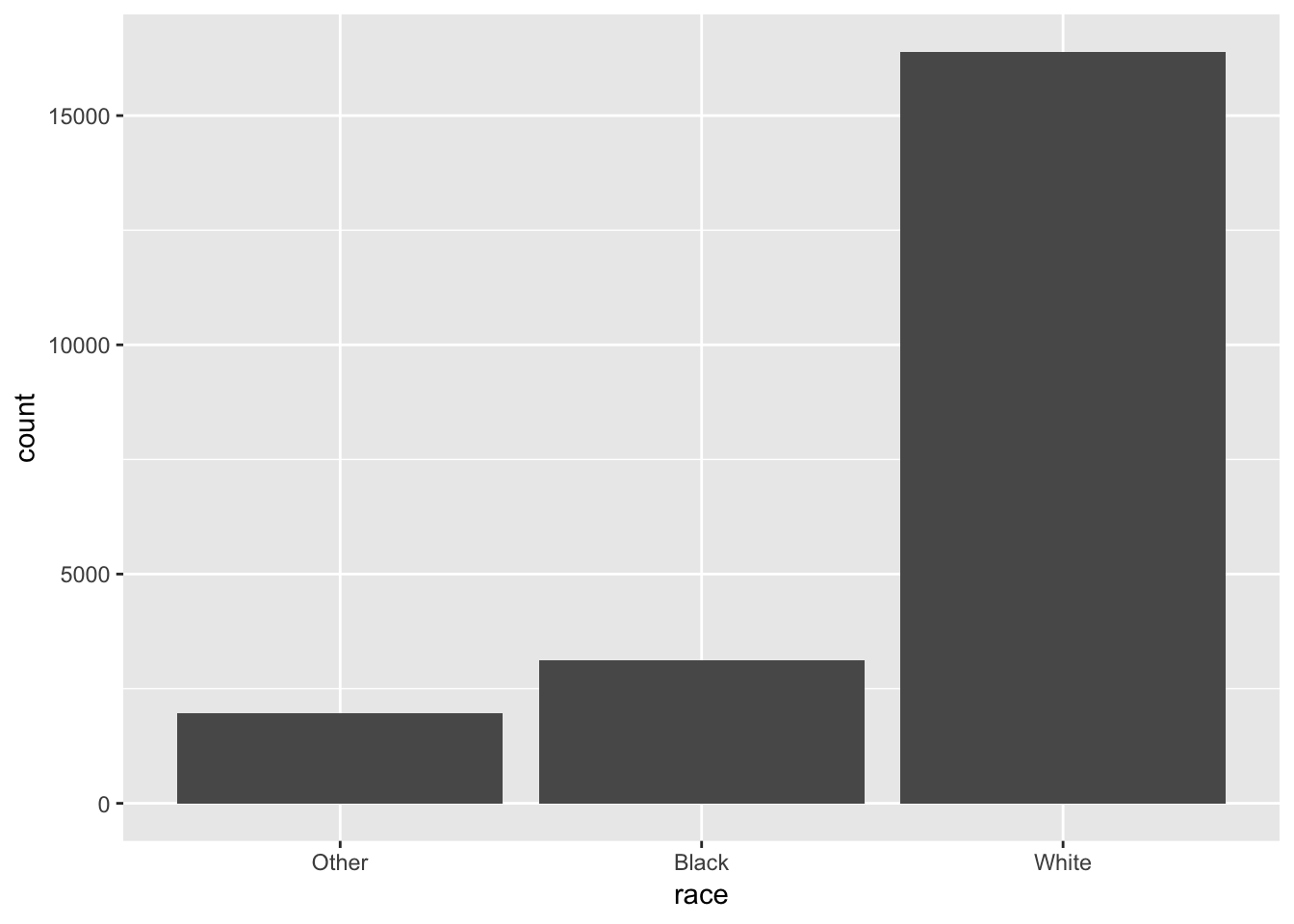Note that `ggplot` drops levels that don’t have any values. `ggplot` has an option to show all levels:

``````ggplot(gss_cat, aes(race)) +
geom_bar() +
scale_x_discrete(drop = FALSE)``````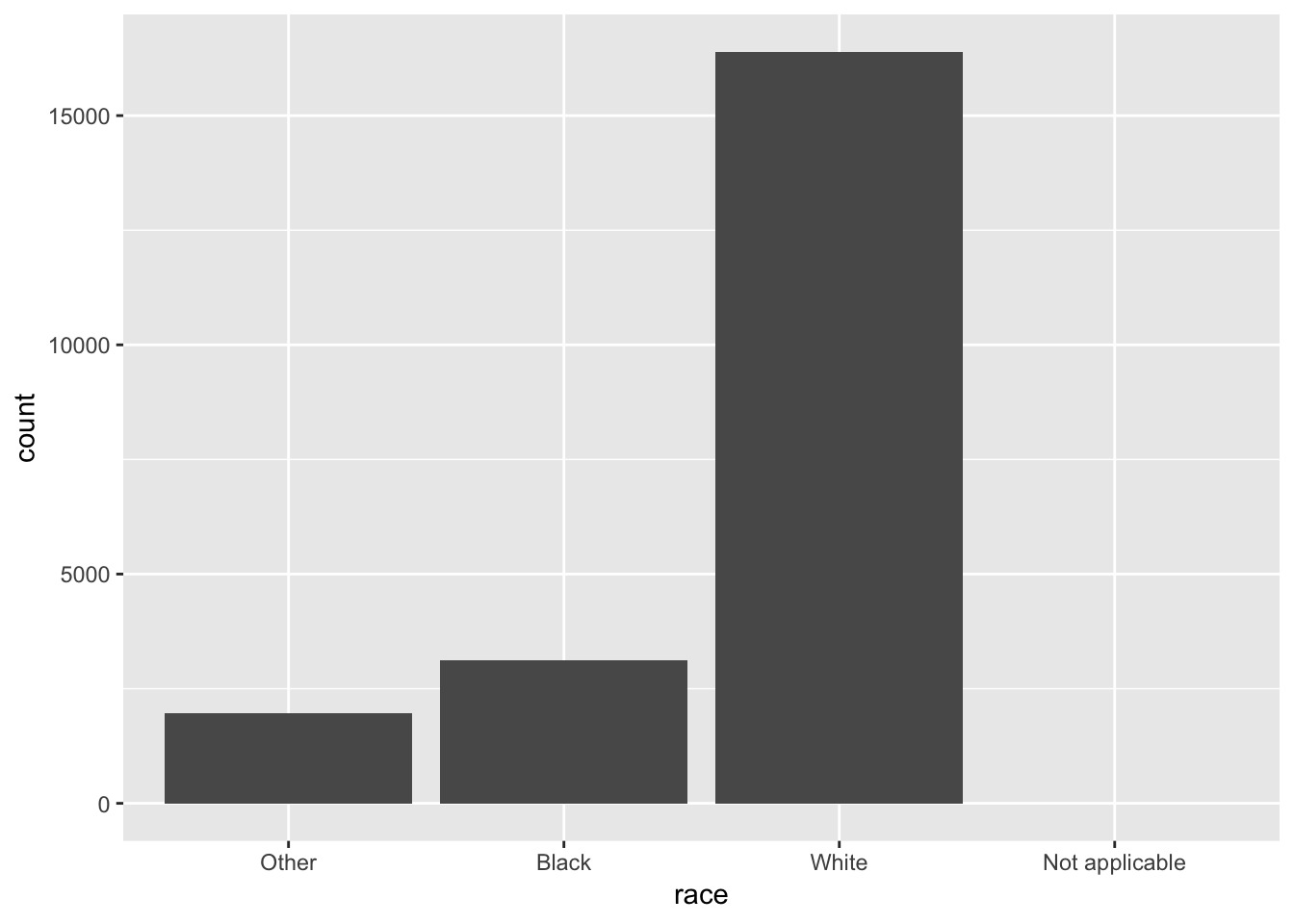## Creating factors

We’ve taken a look at the factors of a dataset, but there may be instances when you need to create the variables yourself.

You could do it this way:

``x1 <- c("Dec", "Apr", "Jan", "Mar")``

This creates a vector of strings (it’s not a `factor`).

But there are some risks.

1. There’s no check on typos.
``````x_typo <- c("Dec", "Apr", "Jam", "Mar")
x_typo``````
``##  "Dec" "Apr" "Jam" "Mar"``
1. It likely doesn’t sort in a useful way.
``sort(x1)``
``##  "Apr" "Dec" "Jan" "Mar"``

Above, it simply sorted the months alphabetically, though it would probably be more helpful to be in month order.

Using a `factor` can address these issues. To create a `factor`, create a list of the levels.

``````month_levels <- c(
"Jan", "Feb", "Mar", "Apr", "May", "Jun",
"Jul", "Aug", "Sep", "Oct", "Nov", "Dec"
)``````

Create a factor by calling `factor()` and defining the `levels` option:

``````f1 <- factor(x1, levels = month_levels)
f1``````
``````##  Dec Apr Jan Mar
## Levels: Jan Feb Mar Apr May Jun Jul Aug Sep Oct Nov Dec``````
``sort(f1)``
``````##  Jan Mar Apr Dec
## Levels: Jan Feb Mar Apr May Jun Jul Aug Sep Oct Nov Dec``````

If you omit the `levels` option, they’ll be taken from the data in alphabetical order.

``factor(x1)``
``````##  Dec Apr Jan Mar
## Levels: Apr Dec Jan Mar``````

Values that are not set in the levels assignment will be silently converted to `NA`.

``````f_typo <- factor(x_typo, levels = month_levels)
f_typo``````
``````##  Dec  Apr  <NA> Mar
## Levels: Jan Feb Mar Apr May Jun Jul Aug Sep Oct Nov Dec``````

## Changing `factor` order

You can take a look at all of the functions in the `forcats` package here.

When defining levels, their order matters. Taking a look at `month_levels` that we assigned above, we will see that the order in which we assigned the levels is retained.

It can be useful to change the order of factor levels for visualizations.

We’ll go over `summarise` and `group_by` in detail next week, but let’s use them here to quickly construct something we can use for our visualization example.

``````relig_summary <- gss_cat %>%
group_by(relig) %>%
summarise(
age = mean(age, na.rm = TRUE),
tvhours = mean(tvhours, na.rm = TRUE),
n = n()
)

ggplot(relig_summary, aes(tvhours, relig)) + geom_point()``````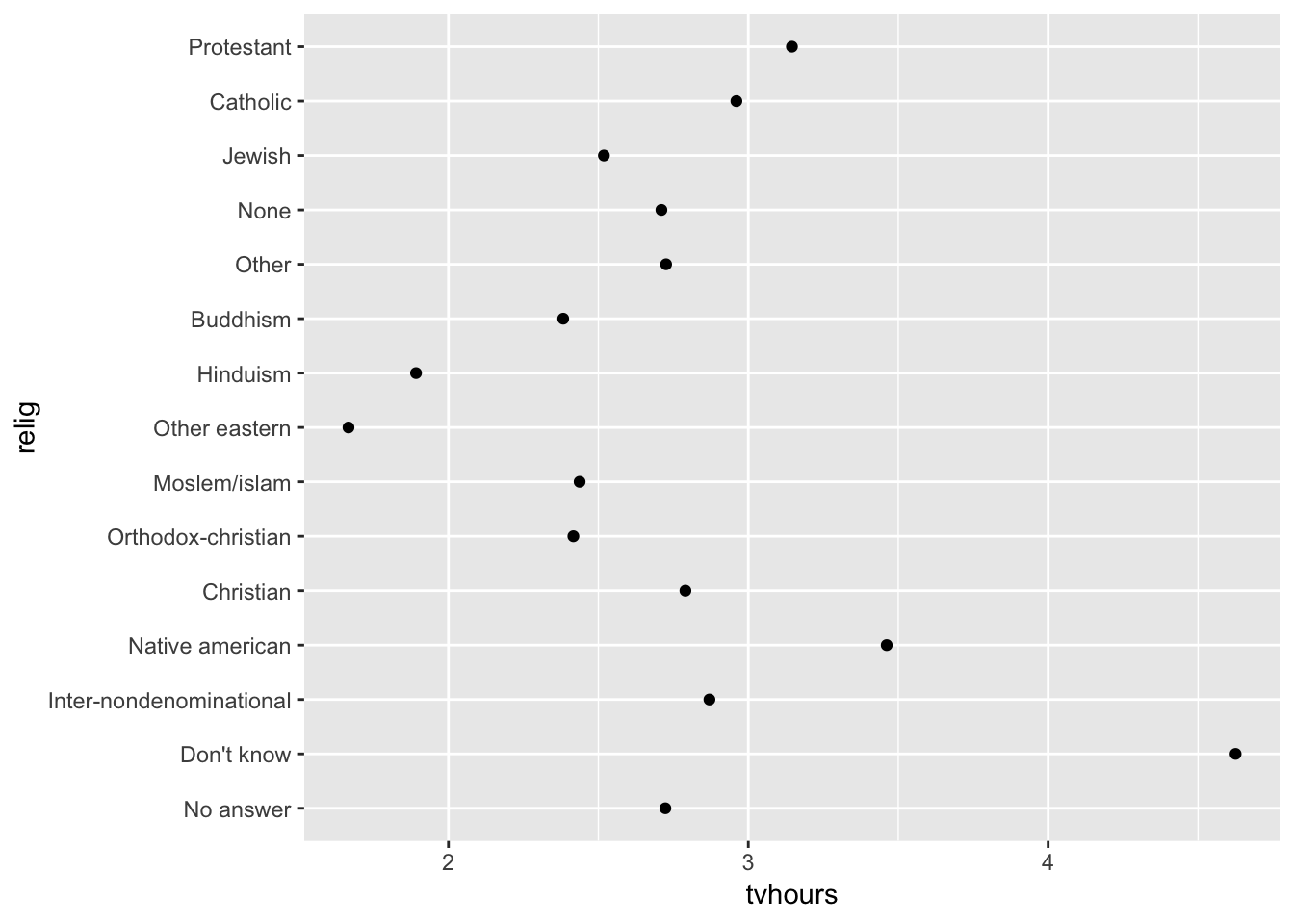There’s not much of a pattern here that we can see. Let’s reorder the factor levels according to `tvhours` using `fct_reorder` from the `forcats` package.

``````relig_summary %>% # Call the dataset that you want to use
# Redefine the levels of the `relig` factor variable
mutate(relig = fct_reorder(relig, tvhours)) %>%
ggplot(aes(tvhours, relig)) +
geom_point()``````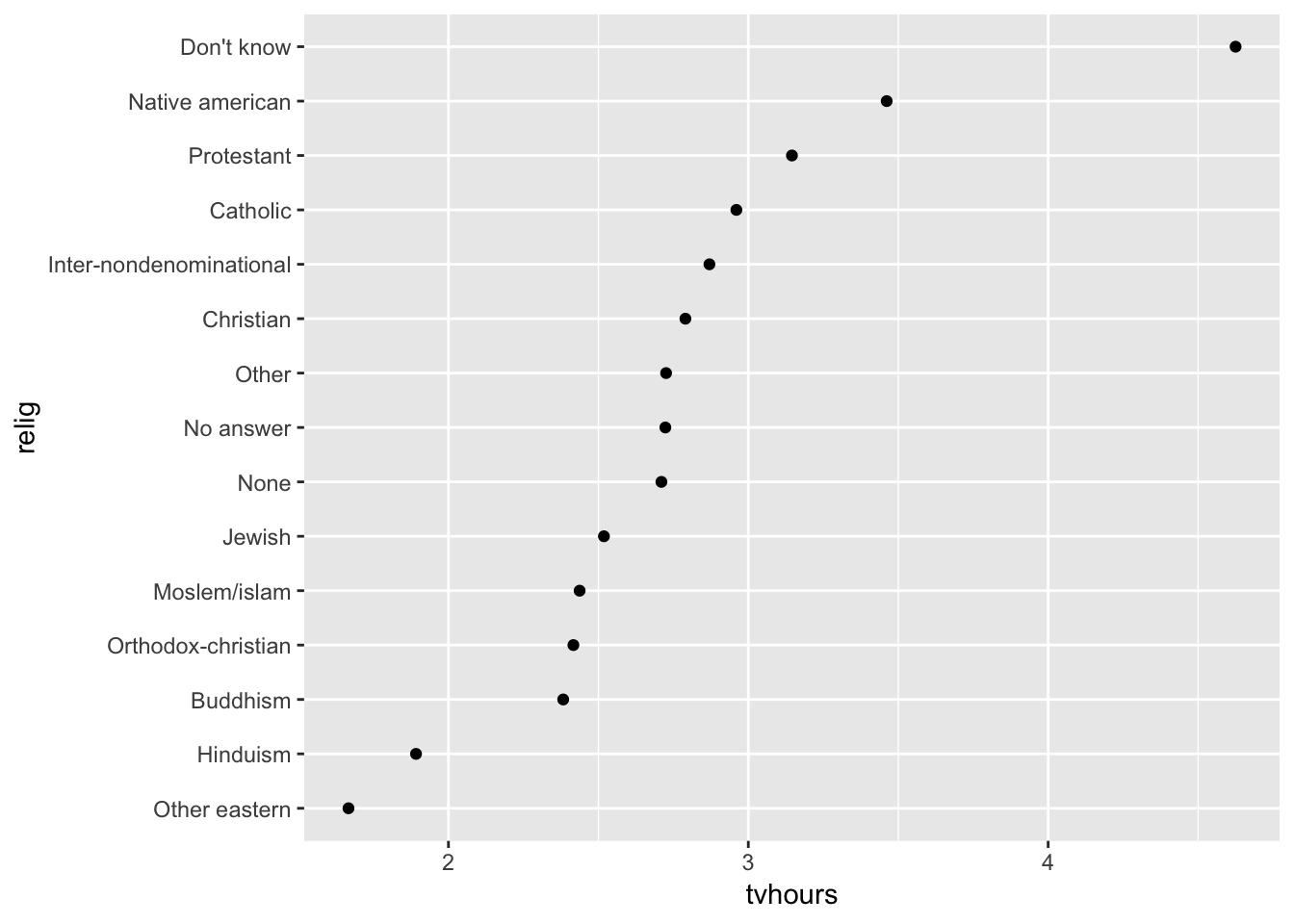We can see from this example that `fct_reorder` reorders the order of factors according to another variable.

Think before reordering – some categorical variables already have a principaled order. In the following example, it wouldn’t make sense to reorder income levels by increasing age.

``````rincome_summary <- gss_cat %>%
group_by(rincome) %>%
summarise(
age = mean(age, na.rm = TRUE),
tvhours = mean(tvhours, na.rm = TRUE),
n = n()
)

ggplot(rincome_summary, aes(age, fct_reorder(rincome, age))) + geom_point()``````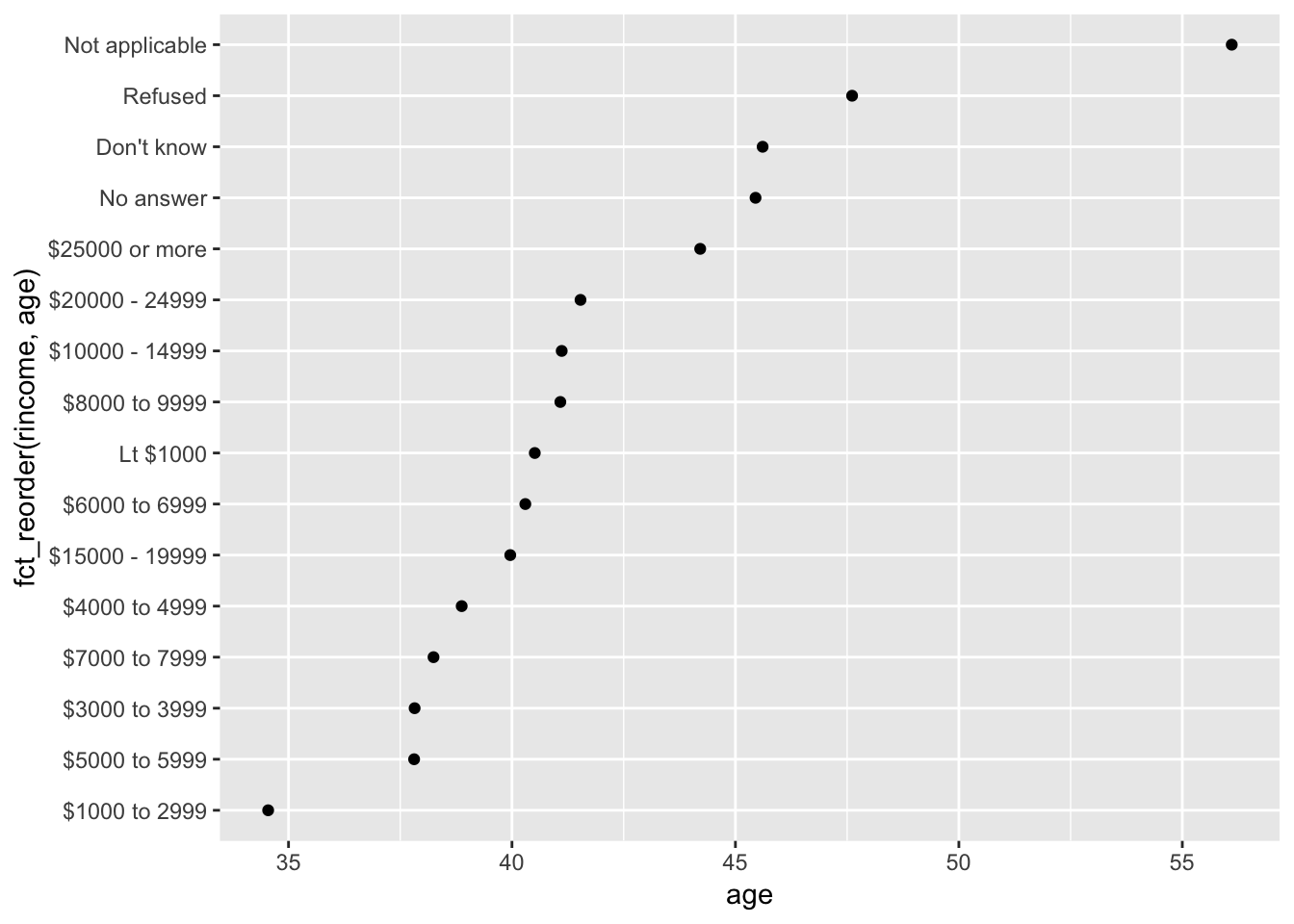Since the levels of the `rincome` variable have a meaningful order, they should probably stay that way.

``levels(rincome_summary\$rincome)``
``````##   "No answer"      "Don't know"     "Refused"        "\$25000 or more"
##   "\$20000 - 24999" "\$15000 - 19999" "\$10000 - 14999" "\$8000 to 9999"
##   "\$7000 to 7999"  "\$6000 to 6999"  "\$5000 to 5999"  "\$4000 to 4999"
##  "\$3000 to 3999"  "\$1000 to 2999"  "Lt \$1000"       "Not applicable"``````

Though we may want to reverse them using `fct_rev()`, so that people making the most money are highest on the y-axis.

``ggplot(rincome_summary, aes(age, fct_rev(rincome))) + geom_point()``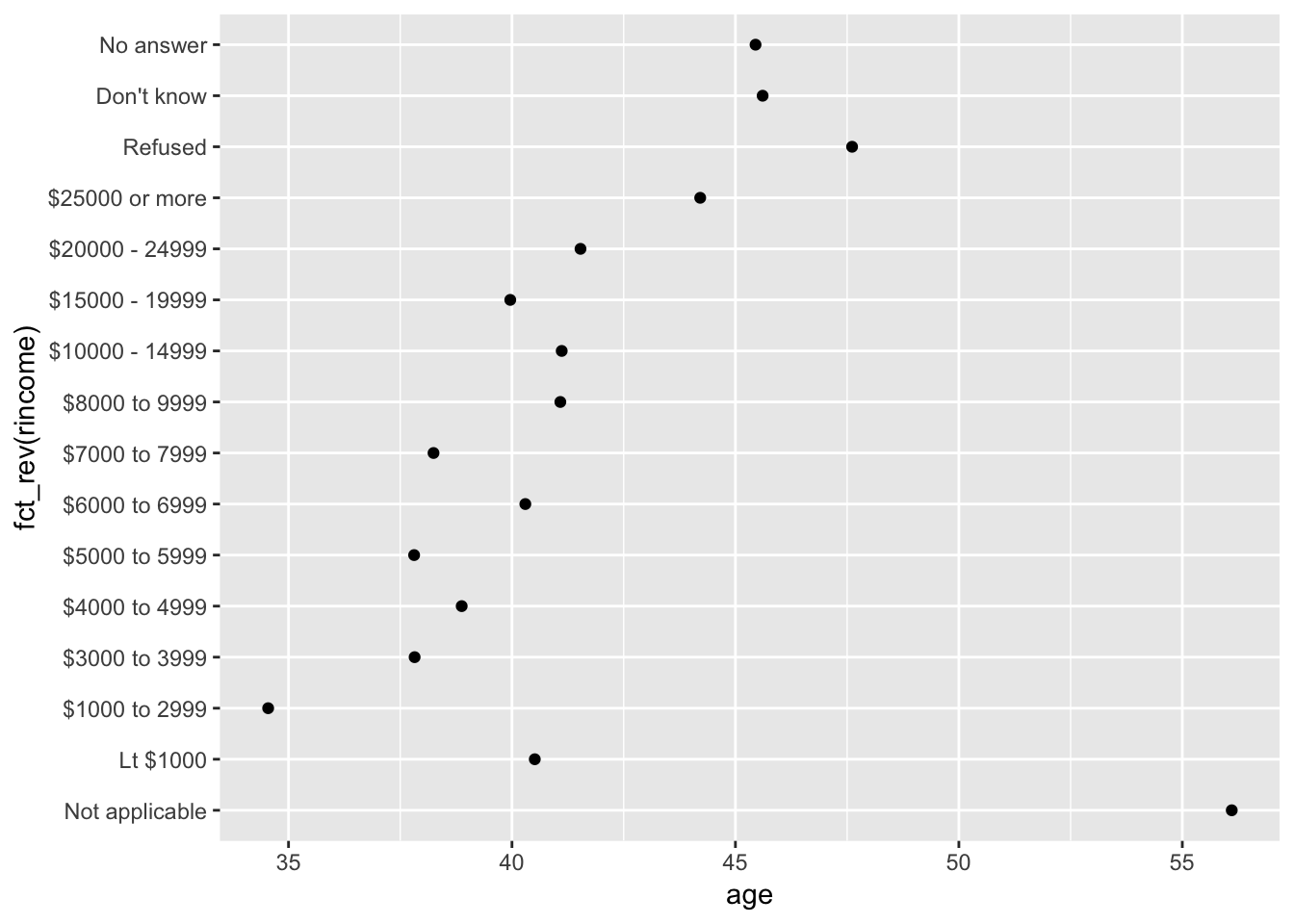It could also make sense to move the “Not applicable” to a spot that makes more sense. For this, we’ll use `fct_relevel`. Instead of designating a second variable to define the order, `fct_relevel` takes any levels that you want to move to the front of the list.

``levels(fct_relevel(rincome_summary\$rincome, "Not applicable"))``
``````##   "Not applicable" "No answer"      "Don't know"     "Refused"
##   "\$25000 or more" "\$20000 - 24999" "\$15000 - 19999" "\$10000 - 14999"
##   "\$8000 to 9999"  "\$7000 to 7999"  "\$6000 to 6999"  "\$5000 to 5999"
##  "\$4000 to 4999"  "\$3000 to 3999"  "\$1000 to 2999"  "Lt \$1000"``````
``# (Note that this isn't saving it in that order in the dataset, just printing it)``
``````rincome_summary %>%
mutate(rincome = fct_relevel(rincome, "Not applicable"),
rincome = fct_rev(rincome)) %>%
ggplot(aes(age, rincome)) +
geom_point()``````